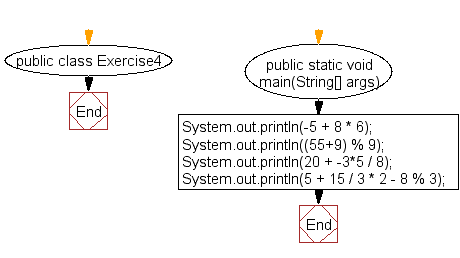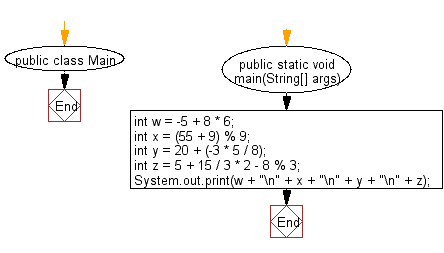﻿ Java exercises: Print the result of the specified operations - w3resource# Java Exercises: Print the result of the specified operations

## Java Basic: Exercise-4 with Solution

Write a Java program to print the result of the following operations.

Pictorial Presentation:Sample Solution:

Java Code:

``````public class Exercise4 {

public static void main(String[] args) {
System.out.println(-5 + 8 * 6);
System.out.println((55+9) % 9);
System.out.println(20 + -3*5 / 8);
System.out.println(5 + 15 / 3 * 2 - 8 % 3);
}

}
```
```

Sample Output:

```43
1
19
13
```

Flowchart:Sample Solution:

Java Code:

``````public class Main {

public static void main(String[] args) {
int w = -5 + 8 * 6;
int x = (55 + 9) % 9;
int y = 20 + (-3 * 5 / 8);
int z = 5 + 15 / 3 * 2 - 8 % 3;

System.out.print(w + "\n" + x + "\n" + y + "\n" + z);
}
}
```
```

Flowchart:Java Code Editor:

What is the difficulty level of this exercise?

Test your Programming skills with w3resource's quiz.

﻿

## Java: Tips of the Day

countOccurrences

Counts the occurrences of a value in an array.

Use Arrays.stream().filter().count() to count total number of values that equals the specified value.

```public static long countOccurrences(int[] numbers, int value) {
return Arrays.stream(numbers)
.filter(number -> number == value)
.count();
}
```

Ref: https://bit.ly/3kCAgLb